Courses

# Test: Squares And Square Roots- 3

## 40 Questions MCQ Test Quantitative Aptitude for GMAT | Test: Squares And Square Roots- 3

Description
This mock test of Test: Squares And Square Roots- 3 for Quant helps you for every Quant entrance exam. This contains 40 Multiple Choice Questions for Quant Test: Squares And Square Roots- 3 (mcq) to study with solutions a complete question bank. The solved questions answers in this Test: Squares And Square Roots- 3 quiz give you a good mix of easy questions and tough questions. Quant students definitely take this Test: Squares And Square Roots- 3 exercise for a better result in the exam. You can find other Test: Squares And Square Roots- 3 extra questions, long questions & short questions for Quant on EduRev as well by searching above.
QUESTION: 1

### (8.01)2 + ? = (8.97)2 What will approximately come in place of question mark?

Solution: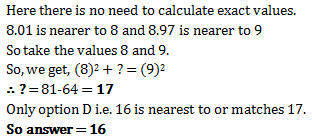QUESTION: 2

### The square of 23 is :

Solution: Yes the correct option is A because..... 23×23=529.....
QUESTION: 3

### The square of which of the following would be even number?

Solution:
Since the square of an odd natural number is odd and that of an even number is an even number.
∴    (i)  The square of 431 is an odd number
(∵  431 is an odd number)
(ii)   The square of 2826 is an even nnumber.
(∵ 2826 is an even number)
(iii) The square of 7779 is an odd number
(∵ 7779 is an odd number)
(iv)  The square of 131 is an odd nnumber.
(∵ 82004 is an odd number)
QUESTION: 4

The square of which of the following would be odd number?

Solution:

Squares of odd numbers are always odd

QUESTION: 5

Which of the following is a perfect square ?

Solution: 81 is the square of 9..... 9 × 9 = 81
QUESTION: 6

What will be the one digit in the square of 1234

Solution:

One digit will be 6 because 1234
4, is at he last and 4² = 16.
So, 6 is at the last.

QUESTION: 7

What will be the number of zeros in the square of 400 ?

Solution:
QUESTION: 8

The perfect square number between 30 and 40 is  :

Solution:

Since 35,32,39 are not perfect squares . hence the only perfect square between 30 and 40 is 36

QUESTION: 9

By what least number should we multiply 1008 to make it a perfect square?

Solution: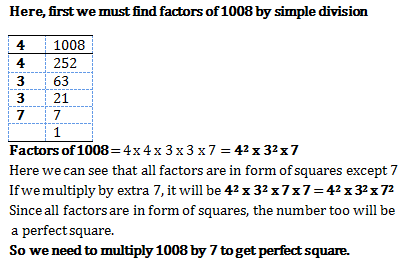QUESTION: 10

Sum of squares of two numbers is 145. If square root of one number is 3, find the other number.

Solution: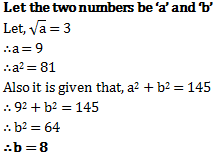QUESTION: 11

Which of the following number would have digit 1 at units place ?

Solution:
QUESTION: 12

How many natural numbers lie between 92 and 102?

Solution:

Between 92 and 102

Here, n = 9 and n + 1 =10

:. Natural number between 92 and 102  are (2 x n) or 2 x 9, i.e. 18. hence,correct option is (c).

QUESTION: 13

How many non square numbers lie between 11and 122?

Solution:

Between 112 and 122

Here, n = 11 and n + 1 = 12

∴ Natural numbers between 112 and 122 are (2 × n) or 2 × 11, i.e. 22.

QUESTION: 14

Which of the following are the factors of ac+ ab + bc + a2

Solution:

The question should be a2+ac+bc+ab
=a(a+c)+b(a+c)=(a+c)(a+b)

QUESTION: 15

How many numbers lie between square of 12 and 13?

Solution:

The number of non square numbers
between n² and ( n + 1 )² is 2n
Here,
n = 12,
n + 1 = 13
Therefore ,
Number of natural numbers lie
between 12² and 13² = 2 × 12
= 24

QUESTION: 16

Which of the following arc the factors of 1 – x2?

Solution:
QUESTION: 17

What is the number of digits in the square root of 390625?

Solution:

Square root of 390625=625.Hence its a 3 digit number

QUESTION: 18

The square root of 6400 is

Solution: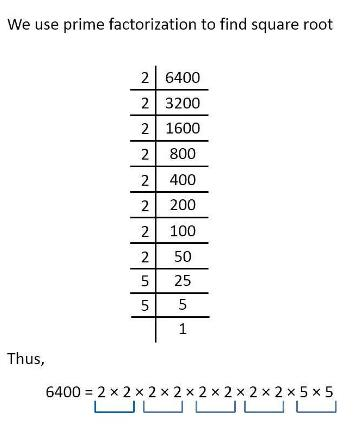Therefore, the square root is 80

QUESTION: 19

By which smallest number 90 must be multiplied so as to make it a perfect square ?

Solution:

By prime factorisation we have 90=2*3*3*5, so the least number by which 90 should be multiplied is 2*5=10

QUESTION: 20

By which smallest number 48 must be divided so as to make it a perfect square ?

Solution:

By Prime factorisation, we have 48=2*2*2*2*3. We have two pairs of 2 but no pair of 3. Hence 48 must be divided by 3 to make it a perfect square.

QUESTION: 21

Which smallest number should be added to 80 so as to make it a perfect square ?

Solution:
QUESTION: 22

What could be the possible “one’s digit” of the square root of 625?

Solution: 625 is square root of 25 and one's digit of 25 is 5
QUESTION: 23

Which is the smallest three-digit perfect square?

Solution: Yes as the the smallest 3 digit no. is 100 and it is the square of 10 so also a perfect square.
QUESTION: 24

Which is the greatest three-digit perfect square?

Solution:
QUESTION: 25

Which is the greatest 4-digit perfect square?

Solution:

By division method we find the root of 9999 and we get the remainder 198.So subtracting 198 from 9999 we get 9801 as the greatest 4-digit perfect square.

QUESTION: 26

Which is the smallest 4-digit perfect square?

Solution:

Apply division method on 1000, gives 3*3=9 leaves remainder 1 .Then get the two zeros, which makes it 100 then you find the next number ie,62*2=124 and then subtract 100 from 124 you get 24 which is to be added in 1000. Hence it’s 1024

QUESTION: 27

What will be the number of digits in the square root of 25600?

Solution:
QUESTION: 28

What will be the number of digits in the square root of 1296?

Solution:
QUESTION: 29

The square root of 12.25 is _______________ .

Solution:
QUESTION: 30

What is the length of the side of a square whose area is 441 cm2 ?

Solution: We know area of a square =a^2 given area =441cm^2 so a^2=441cm2 a^2=(21cm)^2 so a=21...length of square =21cm
QUESTION: 31

In a right angle triangle ABC, right angled at B, AB = 6cm, BC = 8cm ,then AC = ____ .

Solution:

We have (AB)2+(BC)=( AC)2 which is (AC)= 36+64 . Hence AC = 10cm.

QUESTION: 32

Which least number should be subtracted from 629 so as to get a perfect square ?

Solution:
QUESTION: 33

The square root of 1.21 is

Solution: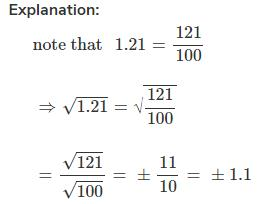QUESTION: 34

What is the smallest square number which is divisible by each of the numbers 6,9 and 15 ?

Solution:

LCM of 6,9 and 15 is 90. So any multiple of 90 will be divisible by 6,9 and 15. The only square number in the options is 900, so 900 is the smallest square number which is divisible by 6,9 and 15.

QUESTION: 35

The square of 1.2 is

Solution:
QUESTION: 36

The square root of 169 is

Solution:

13 is the square root of 169.

QUESTION: 37

What is the length of the diagonal of a rectangle having dimensions 3 cm and 4 cm?

Solution: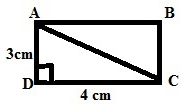Here let us consider ABCD is a rectangle given in the figure.

DC = 4 cm (Length)

To Find:
AC (Diagonal)

We know that Triangle ADC is a right triangle, right angled at D. So by using Pythagorus Theorem

Length^2 + Breadth^2 = Hypotenuse^2     (diagonal)

=> 4^2 + 3^2 = AC^2

=> 16 + 9 = AC^2

=> 25 = AC^2

=> AC = √25 = 5

Therefore the length of the diagonal AC is 5 cm.
QUESTION: 38

What will be the length of third side of a right angled triangle whose hypotenuse is 5cm and one of the side is 3 cm ?

Solution:

By using Pythagoras theorem
H= P+ B2
Third side =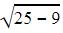= 4cm

QUESTION: 39

Which of the following is not a perfect square ?

Solution:
QUESTION: 40

What is the difference between 1500 and nearest perfect square number smaller than 1500?

Solution:

We know that 402 = 1600

So we must start finding squares of numbers less than 40

Take 39 first

402 = 1600, :. 1600 -1500 = 100

392 = 1521,  :.1521 -1500 = 21

382= 1444, .: 1500 -1444 = 56

Thus nearest perfect square is 392.

But there is no option of difference of 21.

so we'll go the next available nearest possible that is 1444 i.e 382

So answer is 1500-1444 = 56